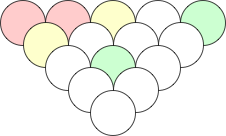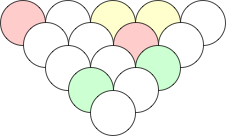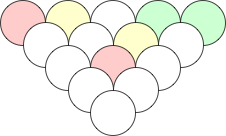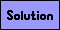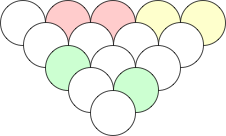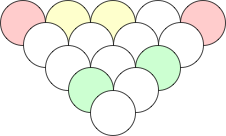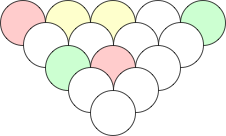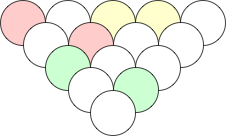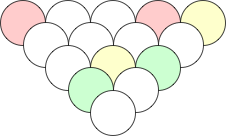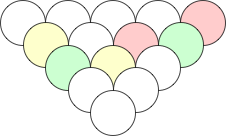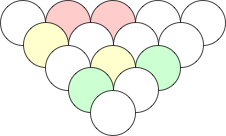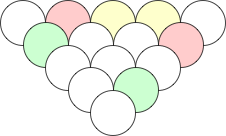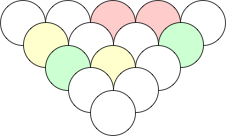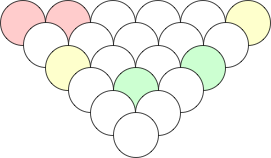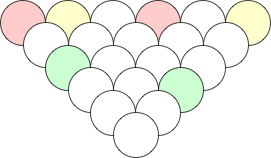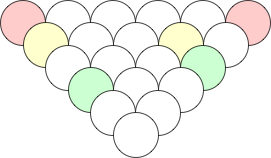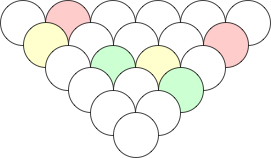Grape Puzzles

Put a positive number in each grape. Each number in the top row of grapes is a single digit. Each number not the top row is the sum of the numbers in the two grapes just above it. If two grapes contain the same number, they are colored the same color. An example is shown below: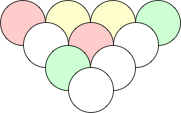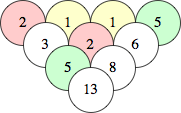Each puzzle below has a unique solution.